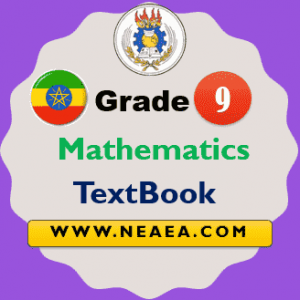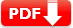# Ethiopian Grade 9 Mathematics Textbook For Students [PDF] Download

Ethiopian Grade 9 Mathematics Textbook For Students [PDF] Download: The Maths students textbook is provided by the MoE Ethiopia free for all school students. The Federal Democratic Republic of Ethiopia Ministry of Education is designed this book according to the new educational framework defined by the Ministry.

The book has a total of 7 units with each unit has its subunit. Students, parents, and teachers can use the ebook for study purposes. Each student should acquire a solid, applicable and extendable mathematical knowledge and develop the appropriate mathematical skills either to pursue his/her study of preparatory school.## Ethiopian Grade 9 Mathematics Student Textbook

In grade 9 the students acquire and develop solid mathematics knowledge, skills, and attitudes that significantly contribute to the creation of citizens who are conscious of the social, economic, political and cultural realities of Ethiopia.

Unit 1: Polynomial function 1.1 Introduction to polynomial functions

• 1.2 Theorems on polynomials
• 1.3 Zeros of a polynomial function
• 1.4 Graphs of polynomial functions

Unit 2: Exponential and logarithmic functions

• 2.1 Exponents and logarithms
• 2.1.1 Exponents
• 2.1.2 Logarithms
• 2.2 The exponential functions and their graphs
• 2.3 The logarithmic functions and their graphs
• 2.4 Equations involving exponents and logarithms
• 2.5 Applications of exponential and logarithmic functions

Unit 3: Solving inequalities 3.1 Systems of linear inequalities involving absolute value

• 3.1 Systems of linear inequalities involving absolute value
• 3.2 Systems of linear inequalities in two variables
• 3.3 Quadratic inequalities

Unit 4: Coordinate geometry

• 4.1 Distance between two points
• 4.2 Division of a line segment
• 4.3 Equation of a line
• 4.4 Parallel and perpendicular lines

Unit 5: Trigonometric functions

• 5.1 Basic trigonometric functions
5.1.1 The sine, cosine and tangent functions
5.1.2 Trigonometric values of angles
5.1.3 Graphs of the sine, cosine and tangent functions.
• 5.2 The reciprocal functions of the basic trigonometric functions
• 5.3 Simple trigonometric identities
• 5.4 Real life application problems

Unit 6: Plane geometry

• 6.1 Theorems on triangles
• 6.2 Special quadrilaterals
• 6.3 More on circles
• 6.4 Regular polygons

Unit 7: Measurement

• 7.1 Revision on surface areas and volumes of prisms and cylinders
• 7.2 Pyramids, cones and spheres
• 7.3 Frustums of pyramids and cones
• 7.4 Surface area and volumes of composed solids.

 Country Ethiopia Publisher MoE, Ethiopia Class Grade 9 Subject Grade 9 Mathematics Type TextBook Group General System Total Units 7 Total Pages 235 TextBook Version 1.0 Download Link